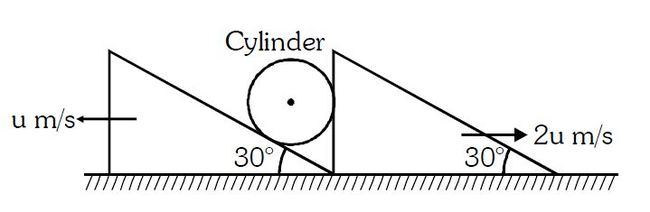# Constraint motion of cylinder!System is shown in the figure. Assume that cylinder remains in contact with the two wedges. If the velocity of cylinder is $u\sqrt n$. Find the value of $n$.

×